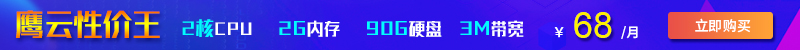这篇文章给大家介绍了“新手学习Python有哪些关键字要掌握”的相关知识，讲解详细，步骤过程清晰，对大家进一步学习和理解“新手学习Python有哪些关键字要掌握”有一定的帮助，希望大家阅读完这篇文章能有所收获。下面就请大家跟着小编的思路一起来学习一下吧。

## 1 and、or、not

and、or、not关键字都是逻辑运算符，用法如下：

and：如果两个语句都返回True，则返回值将仅为True，否则它将返回False。

or：如果其中一条语句返回True，则返回值为True，否则它将返回False。

not：如果语句不是True，则返回值为True，否则返回False。

```x1 = (5 > 3 and 5 < 10) x1 x2 = (5 > 3 or 5 > 10)
x2

x3 = False
not x3
```## 2 if、elif、else

if、elif、else主要用于条件语句，用法如下：

if：用于创建条件语句（if语句），并且仅当条件为True时，才允许执行if代码块。

elif：在条件语句（if语句）中使用，是else if的缩写。

else：在条件语句（if语句）中使用，并确定在if条件为False时该执行的代码。

```def func(x):
if x < 18: print("未成年") elif x < 30: print("青年") else: print("中老年") func(25) ``````def func1(x):
try:
100//x
except:
print("ZeroDivisionError: division by zero(除数不能是0)")
else:
print(f"程序计算结果是{str(100//x)}")

func1(10)
func1(0)
```## 3 for、while

for、while主要用于定义一个循环，用法如下：

for：用于创建一个for循环，它可以用来遍历序列，例如列表，元组等。

while：用于定义while循环，while循环将继续，直到while的条件为False。

```name_list = ["张三","李四","王五"]

for name in name_list:
print(name)
``````x = 0
while x<9: print(x) x += 1 ```## 4 True、False

True、False是比较操作返回的结果，用法如下：

True：关键字True与1相同。

False：关键字False与0相同。

```print(9 > 6)
print(6 in [11,6,33])
print(5 is 5)
print(5 == 5)
print(5 == 5 and 7 == 7)
print(5 == 5 or 6 == 7)
print(not(5 == 7))
``````print(9 < 6) print(6 in [11,88,33]) print(15 is 5) print(5 != 5) print(5 < 5 and 7 == 7) print(5 <= 3 or 6 >= 7)
print(not(5 == 5))
```## 5 continue、break

continue、break主要用在for循环和while循环中，用法如下：

continue：continue关键字用于在for循环（或while循环）中结束当前迭代，并继续进行下一个迭代。

break：break关键字用于中断for循环或while循环。

```for i in range(10):
if i <= 5: continue print(i) ``````x = 0
while x < 10: if x == 5: break x += 1 print(x) ```## 6 pass

pass语句用作将来代码的占位符。当执行pass语句时，不会有任何影响，只是占位作用代表空白代码，但是，如果你不写任何东西，就会报错。循环，函数定义，类定义或if语句中不允许使用空代码，则可以使用pass。## 7 try、except、finally、raise

try、except、finally、raise都是与异常有关的关键词，用法如下：

try：在try…except块中使用，它定义了一个代码块，并在没有问题的情况下执行块。如果包含任何错误，可以为不同的错误类型定义不同的块。

except：在try… except块中使用。 如果try块引发错误，并在有问题的情况下执行对应的代码块。

finally：在try…except块中使用。它定义了一个代码块，当try…except…else块结束时，该代码块将运行。无论try块是否引发错误，都将执行finally代码块。

raise：raise关键字用于引发异常，可以定义引发哪种错误，以及向用户显示错误信息。

```def func(x):
try:
100 // x
except:
print("ZeroDivisionError: division by zero(除数不能是0)")
else:
print(f"结果是：{str(100 // x)}")
finally:
print("无论如何，都会执行！")

func(10)
func(0)
``````x = 15

if x < 18: raise Exception("不好意思，你的年龄小于18岁，未成年") ```## 8 import、from、as

import、from、as均与模块的导入有关，用法如下：

import：用于导入模块。

from：用于从模块中导入指定的部分，按需要导入指定子类或函数，减少不必要的资源浪费。

as：用于创建别名。

```import openpyxl
import pandas as pd
```

## 9 def、return

def、return均与函数有关的关键字，用法如下：

def：用于创建（或定义）一个函数。

return：用于结束所定义的函数，并返回值。

```def func1():
print("关注公众号：数据分析与统计学之美")

func1()
``````def func2(x,y):
return x + y

func2(x=2,y=8)
```## 10 class

class关键字用于创建（或定义）一个类。

```class Person:
name = "张三"
age = 18

p = Person()
p.name,p.age
```## 11 lambda

lambda关键字用于创建一个 “匿名函数”。

```x = lambda a: a + 8
x(2)

y = lambda a,b: a + b
y(1,1)

z = lambda a,b,c: a * c + b
z(2,5,5)
```## 12 del

```x = 1
del x
print(x)
``````x = ["张三","李四","王五"]
del x
print(x)
```## 13 global、nonlocal

global关键字用于创建一个全局变量。nonlocal关键字用于声明一个非局部变量，用于标识外部作用域的变量。

```# 定义一个函数:
def func():
global x
x = "函数中的变量"
# 执行函数:
func()
# x定义在函数中，按说这里打印x会报错，我们看看
print(x)
```## 14 in、is

in、is这两个关键字大家一定要区别开来，用法如下：

in：一方面可以用于检查序列（list，range，字符串等）中是否存在某个值。也可以用于遍历for循环中的序列。

is：用于判断两个变量是否是同一个对象，如果两个对象是同一对象，则返回True，否则返回False。要与== 区别开来，使用==运算符判断两个变量是否相等。

```x = ["张三","李四","王五"]
"张三" in x
# -------------------------
for i in range(3):
print(i)
``````x = 2.0
y = 2.0
x is y
x == y
```## 15 None

None关键字用于定义一个空值（根本没有值），与0，False或空字符串不同。 None是其自身的数据类型（NoneType），只能为None。

```x = None
print(x)

if x:
print("嘻嘻")
else:
print("哈哈")
```## 16 assert

```x = 666

assert x == 666
assert x == 888,"x应该等于666，你的输入有误！"
```## 17 with

with常和open使用，用于读取或写入文件。

```with open("哈哈.txt","r") as f:
```## 18 yield

yield关键字结束一个函数，返回一个生成器，用于从函数依次返回值。

```def f():
yield 5

f()
next(f())
```2021-12-25 17:52:41

2022-06-02 17:21:34

2022-01-17 18:41:49• 联系我们
• 24小时售后：4006784567
• 24小时TEL ：0668-2555666
• 售前咨询TEL：400-678-4567
•官方微信官方微信
Copyright  ©  QY  Network  Company  Ltd. All  Rights  Reserved. 2003-2019  群英网络  版权所有   茂名市群英网络有限公司